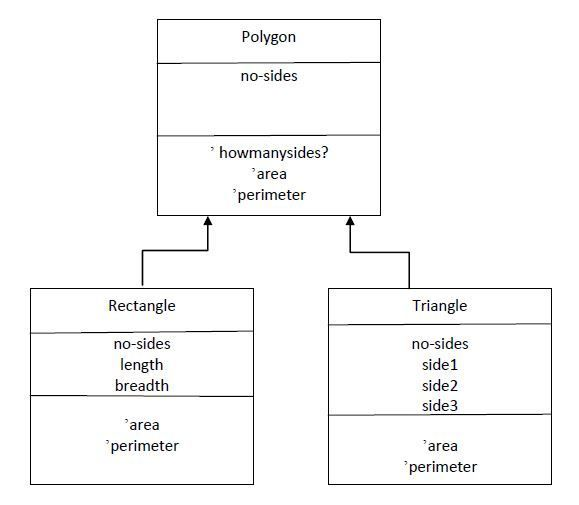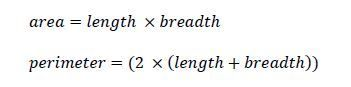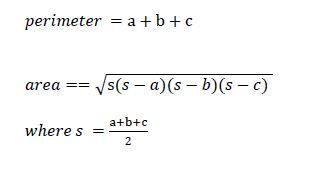Polygon, Rectangle and Triangle Class in PythonPosted by Samath11591 March 25, 2015

Consider three classes polygon, rectangle and triangle, where polygon is the superclass and rectangle and triangle are its subclasses.Complete the implementation of the rectangle class which takes three arguments no-sides (number of sides), breadth and length to create a rectangle object. The rectangle class is a subclass of polygon class. Implement messages area and perimeter for the rectangle class using the formulae given below.Test your implementation using the following expressions;
>>> r1=rectangle(2,4)
>>> r1.area()
8
>>> r1.howmanysides()
4
>>> r1.perimeter()
12
>>>

Complete the implementation of the triangle class which takes four arguments no-sides (number of sides) and the three sides of a triangle a, b and c to create a triangle object. The triangle class is a subclass of polygon class. Implement messages area and perimeter for the triangle class using the formulae given below.Test your implementation using the following expressions;
>>> t1=triangle(5,5,5)
>>> t1.area()
10.825317547305483
>>> t1.howmanysides()
3
>>> t1.perimeter()
15

Code:

```import math
class Polygon:
def __init__(self,nbrsides):
self.nbr_sides = nbrsides

def whoamI(self):
if self.nbr_sides == 3:
return "Triangle"
elif self.nbr_sides == 4:
return "Rectangle"
else: return "Polygon"

def howmanysides(self):
return self.nbr_sides

def area(self):
return "No Area"

def perimeter(self):
return "No Perimeter"

class triangle(Polygon):
def __init__(self,a,b,c):
Polygon.__init__(self, 3)
self.a = a
self.b = b
self.c = c

def area(self):
s = (self.a + self.b + self.c)/2
area = math.sqrt(s*((s-self.a)*(s-self.b)*(s-self.c)))
return area

def perimeter(self):
return self.a + self.b + self.c

class rectangle(Polygon):
Polygon.__init__(self, 4)
self.length = length

def area(self):

def perimeter(self):
return 2 * (self.length + self.breadth)

def main():
tri1=triangle(2,2,2)
rect=rectangle(2,3)
tri2=triangle(3,3,3)
figures = [tri1,rect,tri2]
for fig in figures:
print ("Type of Polygon =>", fig.whoamI())
print ("Sides =", fig.howmanysides())
print ("Area =", fig.area())
print ("Perimeter =", fig.perimeter())
```